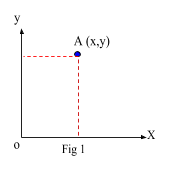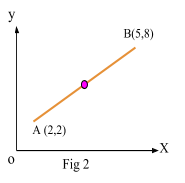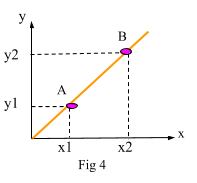# Coordinate Geometry

Contents

## Summary

• Midpoint of a line:$M\quad =\quad (\frac { { x }_{ 1 }\quad +\quad { x }_{ 2 } }{ 2 } ,\quad \frac { { y }_{ 1 }\quad +\quad { y }_{ 2 } }{ 2 } )$
• Distance between two points:$AB\quad =\quad \sqrt { { ({ x }_{ 2 }\quad -\quad { x }_{ 1 }) }^{ 2 }\quad +\quad { ({ y }_{ 2 }\quad -\quad { y }_{ 1 }) }^{ 2 } }$
• Gradient of a straight line$=\quad \frac { { y }_{ 2 }\quad -\quad { y }_{ 1 } }{ { x }_{ 2 }\quad -\quad { x }_{ 1 } }$
• 3 forms of equation of a straight line:
• Gradient Intercept Form:$y\quad =\quad mx\quad +\quad c$
• Gradient Point Form:$y\quad -\quad { y }_{ 1 }\quad =\quad m(x\quad -\quad { x }_{ 1 })$
• Two Point Form:$\frac { y\quad -\quad { y }_{ 1 } }{ { y }_{ 2 }\quad -\quad { y }_{ 1 } } \quad =\quad \frac { x\quad -\quad { x }_{ 1 } }{ { x }_{ 2 }\quad -\quad { x }_{ 1 } }$

#### What is coordinate geometry?

As we know from GCSE’s geometry is basically about how shapes, points, lines all relate to each other. When we add coordinates to it, we are basically describing the location of those shapes, points or lines in space. Coordinate geometry is also known as cartesian geometry.

As shown in Fig 1, the position of a point in a plane is given by an ordered pair of numbers written as (x,y).They are called cartesian coordinates. x and y measure the displacement of the point from two perpendicular axes(ox & oy) intersecting at o, where o is the origin.

#### Midpoint of a line segment

We know that a midpoint of a line is basically the center point of that line.

Generally, it is defined as:

If M is the midpoint of the line joining the points A(x1, y1) and B(x2, y2), then its coordinates are given by:$M\quad =\quad (\frac { { x }_{ 1 }\quad +\quad { x }_{ 2 } }{ 2 } ,\quad \frac { { y }_{ 1 }\quad +\quad { y }_{ 2 } }{ 2 } )$

#### Example #1

Q. Find the midpoint of the line in Fig 2.Solution:

Use the above formula and substitute the values of x and y to work out the midpoint of the line joining A and B.$M\quad =\quad (\frac { { x }_{ 1 }\quad +\quad { x }_{ 2 } }{ 2 } ,\quad \frac { { y }_{ 1 }\quad +\quad { y }_{ 2 } }{ 2 } )$

Put points A(2, 2) and B(5, 8) in above equation:$M\quad =\quad (\frac { 1\quad +\quad 5 }{ 2 } ,\quad \frac { 2\quad +\quad 8 }{ 2 } )$$M\quad =\quad (\frac { 6 }{ 2 } ,\quad \frac { 10 }{ 2 } )$

Ans:$M\quad =\quad (3,\quad 5)$

#### Distance between two points

We want to find the length of PQ where point P and Q are given by the coordinates (4, 7) and (8, 15) respectively.By pythagoras theorem:${ (PQ) }^{ 2 }\quad =\quad { (PR) }^{ 2 }\quad +\quad { (RQ) }^{ 2 }$$=\quad { (8\quad -\quad 4) }^{ 2 }\quad +\quad { (15\quad -\quad 7) }^{ 2 }$$=\quad { 4 }^{ 2 }\quad +\quad 8^{ 2 }$

Hence:$PQ\quad =\quad \sqrt { 80 }$

Ans:$=\quad 4\sqrt { 5 } units$

As shown in Fig 4, to find the gradient (slope) of a straight line, we simply divide the change in y by the change in x between any two points on the line. In this case the points are A and B.We know that slope of a straight line is always constant. The slope basically characterises the direction of the line. We find the gradient using the following formula:

Gradient of a line$=\quad \frac { { y }_{ 2 }\quad -\quad { y }_{ 1 } }{ { x }_{ 2 }\quad -\quad { x }_{ 1 } }$

Remember these points:

1. Note, that it is not important in which order the two points A(x1, y1) and B(x2, y2) are considered for calculations.
2. If AB is parallel to the x axis then the gradient is equal to 0.
3. If AB is perpendicular to x axis then the gradient is undefined.
4. If AB with gradient${ m }_{ 1 }$ is parallel to another line PQ with gradient${ m }_{ 2 }$, then there gradients are equal.${ { (m }_{ 1 }\quad =\quad m }_{ 2 })$
5. If two lines with gradient${ m }_{ 1 }$ and${ m }_{ 2 }$ are perpendicular to each other, then the product of these gradients is equal to -1.${ { (m }_{ 1 }\quad \times \quad m }_{ 2 }\quad =\quad -1)$

#### Example #2

Q. Prove that line$AB\quad y\quad =\quad 2x\quad +\quad 3$ and line$PQ\quad y\quad =\quad \frac { -1 }{ 2 } x\quad +\quad 7$ are perpendicular to each other.

Solution:

Gradient of line AB${ m }_{ 1 }\quad =\quad 2$

Gradient of line PQ${ m }_{ 2 }\quad =\quad \frac { -1 }{ 2 }$

As:${ m }_{ 1 }\quad \times \quad { m }_{ 2 }\quad =\quad -1$

Since:$2\quad \times \quad \frac { -1 }{ 2 } \quad =\quad -1$$-1\quad =\quad -1$

Hence, this proves both lines are perpendicular to each other.

#### Equation of a straight line

Depending upon the given conditions, we can find different forms of the equation of a straight line.

Equation of a line with gradients m and y intercept c is:$y\quad =\quad mx\quad +\quad c$$y\quad -\quad { y }_{ 1 }\quad =\quad m(x\quad -\quad { x }_{ 1 })$$\frac { y\quad -\quad { y }_{ 1 } }{ { y }_{ 2 }\quad -\quad { y }_{ 1 } } \quad =\quad \frac { x\quad -\quad { x }_{ 1 } }{ { x }_{ 2 }\quad -\quad { x }_{ 1 } }$